In addition to the other services for training models, ADS includes a hyperparameter tuning framework called ADSTuner.

ADSTuner supports using several hyperparameter search strategies that plug into common model architectures like sklearn.

ADSTuner further supports users defining their own search spaces and strategies. This makes ADSTuner functional and useful with any ML library that doesn’t include hyperparameter tuning.

First, import the packages:

import category_encoders as ce
import lightgbm
import logging
import numpy as np
import os
import pandas as pd
import pytest
import sklearn
import xgboost

from lightgbm import LGBMClassifier
from sklearn import preprocessing
from sklearn.compose import ColumnTransformer
from sklearn.decomposition import PCA
from sklearn.impute import SimpleImputer
from sklearn.linear_model import SGDClassifier, LogisticRegression
from sklearn.metrics import make_scorer, f1_score
from sklearn.model_selection import train_test_split
from sklearn.pipeline import Pipeline
from sklearn.preprocessing import StandardScaler
from sklearn.feature_selection import SelectKBest, f_classif
from xgboost import XGBClassifier


This is an example of running the ADSTuner on a support model SGD from sklearn:

model = SGDClassifier() ##Initialize the model
X_train, X_valid, y_train, y_valid = train_test_split(X, y)
tuner = ADSTuner(model, cv=3) ## cv is cross validation splits
tuner.search_space() ##This is the default search space
tuner.tune(X_train, y_train, exit_criterion=[NTrials(10)])


ADSTuner generates a tuning report that lists its trials, best performing hyperparameters, and performance statistics with: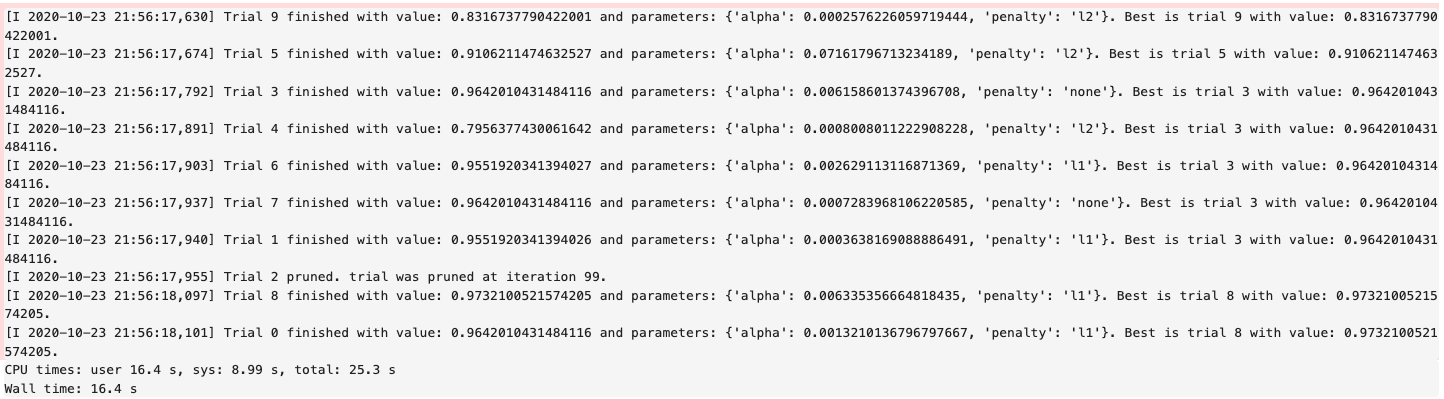You can use tuner.best_score to get the best score on the scoring metric used (accessible as tuner.scoring_name). The best selected parameters are obtained with tuner.best_params and the complete record of trials with tuner.trials

If you have further compute resources and want to continue hyperparameter optimization on a model that has already been optimized, you can use:

tuner.resume()
print('So far the best {} score is {}'.format(tuner.scoring_name, tuner.best_score))
print("The best trial found was number: " + str(tuner.best_index))


ADSTuner has some robust visualization and plotting capabilities:

tuner.plot_best_scores()
tuner.plot_intermediate_scores()
tuner.search_space()
tuner.plot_contour_scores(params=['penalty', 'alpha'])
tuner.plot_parallel_coordinate_scores(params=['penalty', 'alpha'])
tuner.plot_edf_scores()


These commands produce the following plots: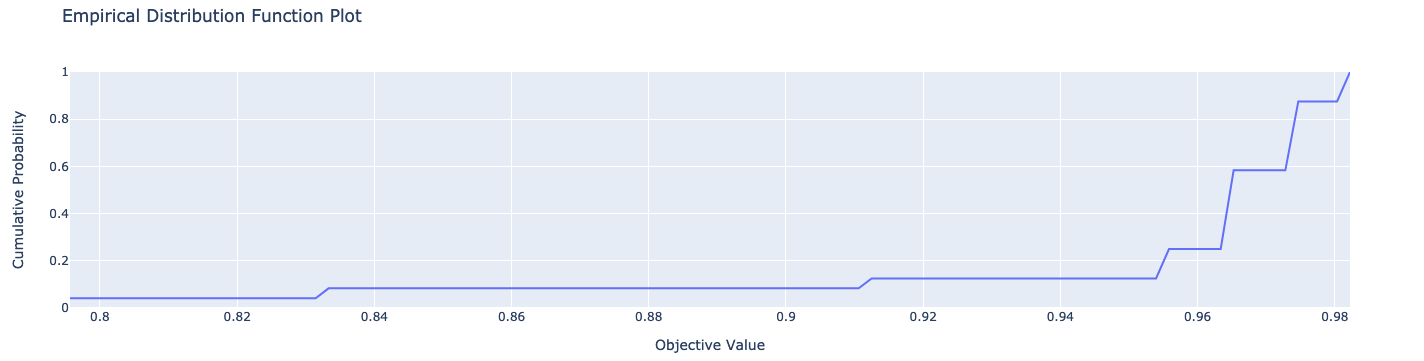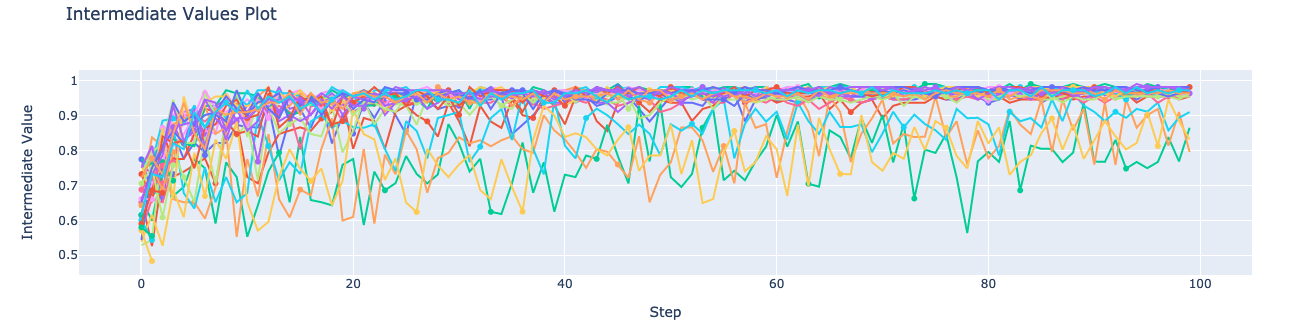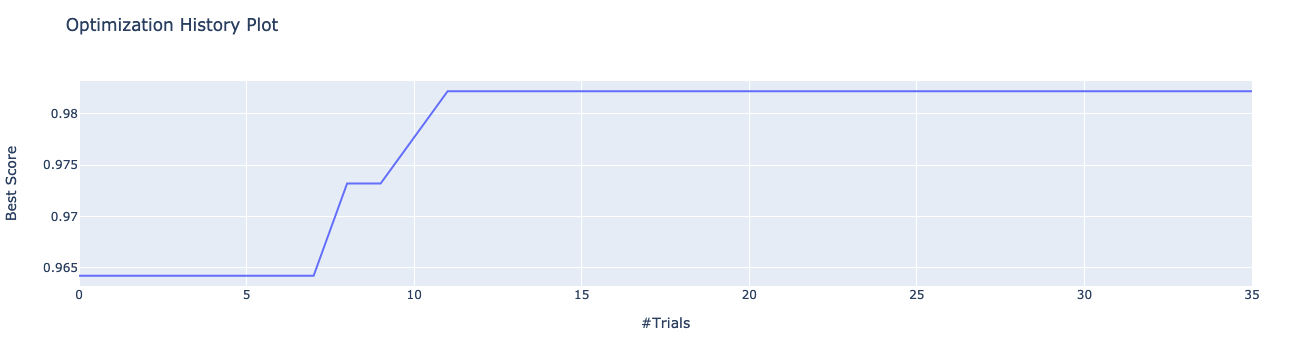ADSTuner supports custom scoring functions and custom search spaces. This example uses a different model:

model2 = LogisticRegression()
strategy = {
'C': LogUniformDistribution(low=1e-05, high=1),
'solver': CategoricalDistribution(['saga']),
'max_iter': IntUniformDistribution(500, 1000, 50)},
scoring=make_scorer(f1_score, average='weighted'),
cv=3)
tuner.tune(X_train, y_train, exit_criterion=[NTrials(5)])


ADSTuner doesn’t support every model. The supported models are:

• ‘Ridge’,

• ‘RidgeClassifier’,

• ‘Lasso’,

• ‘ElasticNet’,

• ‘LogisticRegression’,

• ‘SVC’,

• ‘SVR’,

• ‘LinearSVC’,

• ‘LinearSVR’,

• ‘DecisionTreeClassifier’,

• ‘DecisionTreeRegressor’,

• ‘RandomForestClassifier’,

• ‘RandomForestRegressor’,

• ‘XGBClassifier’,

• ‘XGBRegressor’,

• ‘ExtraTreesClassifier’,

• ‘ExtraTreesRegressor’,

• ‘LGBMClassifier’,

• ‘LGBMRegressor’,

• ‘SGDClassifier’,

• ‘SGDRegressor’

The AdaBoostRegressor model is not supported. This is an example of a custom strategy to use with this model:

model3 = AdaBoostRegressor()
X_train, X_valid, y_train, y_valid = train_test_split(X, y)
tuner = ADSTuner(model3, strategy={'n_estimators': IntUniformDistribution(50, 100)})
tuner.tune(X_train, y_train, exit_criterion=[TimeBudget(5)])


Finally, ADSTuner supports sklearn pipelines:

df, target = pd.read_csv(os.path.join('~', 'advanced-ds', 'tests', 'vor_datasets', 'vor_titanic.csv')), 'Survived'
X = df.drop(target, axis=1)
y = df[target]

numeric_features = X.select_dtypes(include=['int64', 'float64', 'int32', 'float32']).columns
categorical_features = X.select_dtypes(include=['object', 'category', 'bool']).columns

y = preprocessing.LabelEncoder().fit_transform(y)

X_train, X_valid, y_train, y_valid = train_test_split(X, y, test_size=0.3, random_state=42)

num_features = len(numeric_features) + len(categorical_features)

numeric_transformer = Pipeline(steps=[
('num_imputer', SimpleImputer(strategy='median')),
('num_scaler', StandardScaler())
])

categorical_transformer = Pipeline(steps=[
('cat_imputer', SimpleImputer(strategy='constant', fill_value='missing')),
('cat_encoder', ce.woe.WOEEncoder())
])

preprocessor = ColumnTransformer(
transformers=[
('num', numeric_transformer, numeric_features),
('cat', categorical_transformer, categorical_features)
]
)

pipe = Pipeline(
steps=[
('preprocessor', preprocessor),
('feature_selection', SelectKBest(f_classif, k=int(0.9 * num_features))),
('classifier', LogisticRegression())
]
)

def customerize_score(y_true, y_pred, sample_weight=None):
score = y_true == y_pred
return np.average(score, weights=sample_weight)

score = make_scorer(customerize_score)
pipe,
scoring=score,
strategy='detailed',
cv=2,
random_state=42
)


## Notebook Example: Hyperparameter Optimization with ADSTuner#

Overview:

A hyperparameter is a parameter that is used to control a learning process. This is in contrast to other parameters that are learned in the training process. The process of hyperparameter optimization is to search for hyperparameter values by building many models and assessing their quality. This notebook provides an overview of the ADSTuner hyperparameter optimization engine. ADSTuner can optimize any estimator object that follows the scikit-learn API.

Objectives:

• Introduction

• Synchronous Tuning with Exit Criterion Based on Number of Trials

• Asynchronously Tuning with Exit Criterion Based on Time Budget

• Inspecting the Tuning Trials

• Defining a Custom Search Space and Score

• Changing the Search Space Strategy

• Optimizing a scikit-learn Pipeline()

• References

Important:

Placeholder text for required values are surrounded by angle brackets that must be removed when adding the indicated content. For example, when adding a database name to database_name = "<database_name>" would become database_name = "production".

Datasets are provided as a convenience. Datasets are considered third party content and are not considered materials under your agreement with Oracle applicable to the services. The iris dataset is distributed under the BSD license.

import category_encoders as ce
import lightgbm
import logging
import numpy as np
import os
import pandas as pd
import sklearn
import time

from sklearn import preprocessing
from sklearn.compose import ColumnTransformer
from sklearn.decomposition import PCA
from sklearn.impute import SimpleImputer
from sklearn.linear_model import SGDClassifier, LogisticRegression
from sklearn.metrics import make_scorer, f1_score
from sklearn.model_selection import train_test_split
from sklearn.pipeline import Pipeline
from sklearn.preprocessing import StandardScaler
from sklearn.feature_selection import SelectKBest, f_classif


Introduction

Hyperparameter optimization requires a model, dataset, and an ADSTuner object to perform the search.

ADSTuner() Performs a hyperparameter search using cross-validation. You can specify the number of folds you want to use with the cv parameter.

Because the ADSTuner() needs a search space in which to tune the hyperparameters, you must use the strategy parameter. This parameter can be set in two ways. You can specify detailed search criteria or you can use the built-in defaults. For the supported model classes, ADSTuner provides perfunctoryand detailed search spaces that are optimized for the chosen class of model. The perfunctory option is optimized for a small search space so that the most important hyperparameters are tuned. Generally, this option is used early in your search as it reduces the computational cost and allows you to assess the quality of the model class that you are using. The detailed search space instructs ADSTuner to cover a broad search space by tuning more hyperparameters. Typically, you would use it when you have determined what class of model is best suited for the dataset and type of problem you are working on. If you have experience with the dataset and have a good idea of what the best hyperparameter values are, you can explicitly specify the search space. You pass a dictionary that defines the search space into the strategy.

The parameter storage takes a database URL. For example, sqlite:////home/datascience/example.db. When storage is set to the default value None, a new sqlite database file is created internally in the tmp folder with a unique name. The name format is sqlite:////tmp/hpo_*.db. study_name is the name of this study for this ADSTuner object. Each ADSTuner object has a unique study_name. However, one database file can be shared among different ADSTuner objects. load_if_exists controls whether to load an existing study from an existing database file. If False, it raises a DuplicatedStudyError when the study_name exists.

The loglevel parameter controls the amount of logging information displayed in the notebook.

This notebook uses the scikit-learn SGDClassifer() model and the iris dataset. This model object is a regularized linear model with stochastic gradient descent (SGD) used to optimize the model parameters.

The next cell creates the SGDClassifer() model, initialize san ADSTuner object, and loads the iris data.

tuner = ADSTuner(SGDClassifier(), cv=3, loglevel=logging.WARNING)

[32m[I 2023-07-18 15:40:56,115][0m A new study created in RDB with name: hpo_9933857f-c10a-4ece-9828-434aea4b07c2[0m


Each model class has a set of hyperparameters that you need to optimized. The strategy attribute returns what strategy is being used. This can be perfunctory, detailed, or a dictionary that defines the strategy. The method search_space() always returns a dictionary of hyperparameters that are to be searched. Any hyperparameter that is required by the model, but is not listed, uses the default value that is defined by the model class. To see what search space is being used for your model class when strategy is perfunctory or detailed use the search_space() method to see the details.

The adstuner_search_space_update.ipynb notebook has detailed examples about how to work with and update the search space.

The next cell displaces the search strategy and the search space.

print(f'Search Space for strategy "{tuner.strategy}" is: \n {tuner.search_space()}')

Search Space for strategy "perfunctory" is:
{'alpha': LogUniformDistribution(low=0.0001, high=0.1), 'penalty': CategoricalDistribution(choices=['l1', 'l2', 'none'])}


The tune() method starts a tuning process. It has a synchronous and asynchronous mode for tuning. The mode is set with the synchronous parameter. When it is set to False, the tuning process runs asynchronously so it runs in the background and allows you to continue your work in the notebook. When synchronous is set to True, the notebook is blocked until tune() finishes running. The adntuner_sync_and_async.ipynb notebook illustrates this feature in a more detailed way.

The ADSTuner object needs to know when to stop tuning. The exit_criterion parameter accepts a list of criteria that cause the tuning to finish. If any of the criteria are met, then the tuning process stops. Valid exit criteria are:

• NTrials(n): Run for n number of trials.

• TimeBudget(t): Run for t seconds.

• ScoreValue(s): Run until the score value exceeds s.

The default behavior is to run for 50 trials (NTrials(50)).

The stopping criteria are listed in the ads.hpo.stopping_criterion module.

Synchronous Tuning with Exit Criterion Based on Number of Trials

This section demonstrates how to perform a synchronous tuning process with the exit criteria based on the number of trials. In the next cell, the synchronous parameter is set to True and the exit_criterion is set to [NTrials(5)].

tuner.tune(X, y, exit_criterion=[NTrials(5)], synchronous=True)


You can access a summary of the trials by looking at the various attributes of the tuner object. The scoring_name attribute is a string that defines the name of the scoring metric. The best_score attribute gives the best score of all the completed trials. The best_params parameter defines the values of the hyperparameters that have to lead to the best score. Hyperparameters that are not in the search criteria are not reported.

print(f"So far the best {tuner.scoring_name} score is {tuner.best_score} and the best hyperparameters are {tuner.best_params}")

So far the best mean accuracy score is 0.9733333333333333 and the best hyperparameters are {'alpha': 0.04730029773763652, 'penalty': 'none'}


You can also look at the detailed table of all the trials attempted:

tuner.trials.tail()

number value datetime_start datetime_complete duration params_alpha params_penalty user_attrs_mean_fit_time user_attrs_mean_score_time user_attrs_mean_test_score user_attrs_metric user_attrs_split0_test_score user_attrs_split1_test_score user_attrs_split2_test_score user_attrs_std_fit_time user_attrs_std_score_time user_attrs_std_test_score state
0 0 0.846667 2023-07-18 15:40:56.236058 2023-07-18 15:40:57.815838 0 days 00:00:01.579780 0.000269 l2 0.130862 0.025274 0.846667 mean accuracy 0.76 0.88 0.90 0.004218 0.000925 0.061824 COMPLETE
1 1 0.860000 2023-07-18 15:40:57.829067 2023-07-18 15:40:59.349433 0 days 00:00:01.520366 0.000125 l2 0.128980 0.025028 0.860000 mean accuracy 0.80 0.94 0.84 0.003289 0.000634 0.058878 COMPLETE
2 2 0.973333 2023-07-18 15:40:59.358309 2023-07-18 15:41:00.814785 0 days 00:00:01.456476 0.047300 none 0.122376 0.023761 0.973333 mean accuracy 1.00 0.96 0.96 0.002859 0.000554 0.018856 COMPLETE
3 3 0.926667 2023-07-18 15:41:00.823800 2023-07-18 15:41:02.285543 0 days 00:00:01.461743 0.000798 l2 0.122965 0.023687 0.926667 mean accuracy 0.98 0.88 0.92 0.002738 0.000463 0.041096 COMPLETE
4 4 0.846667 2023-07-18 15:41:02.294516 2023-07-18 15:41:03.768919 0 days 00:00:01.474403 0.061982 l2 0.122523 0.023601 0.846667 mean accuracy 0.74 0.94 0.86 0.003231 0.000534 0.082192 COMPLETE

Asynchronously Tuning with Exit Criterion Based on Time Budget

ADSTuner() tuner can be run in an asynchronous mode by setting synchronous=False in the tune() method. This allows you to run other Python commands while the tuning process is executing in the background. This section demonstrates how to run an asynchronous search for the optimal hyperparameters. It uses a stopping criteria of five seconds. This is controlled by the parameter exit_criterion=[TimeBudget(5)].

The next cell starts an asynchronous tuning process. A loop is created that prints the best search results that have been detected so far by using the best_score attribute. It also displays the remaining time in the time budget by using the time_remaining attribute. The attribute status is used to exit the loop.

# This cell will return right away since it's running asynchronous.
tuner.tune(exit_criterion=[TimeBudget(5)])
while tuner.status == State.RUNNING:
print(f"So far the best score is {tuner.best_score} and the time left is {tuner.time_remaining}")
time.sleep(1)

So far the best score is 0.9733333333333333 and the time left is 4.972609996795654
So far the best score is 0.9733333333333333 and the time left is 3.959090232849121
So far the best score is 0.9733333333333333 and the time left is 2.943150043487549
So far the best score is 0.9733333333333333 and the time left is 1.9304382801055908
So far the best score is 0.9733333333333333 and the time left is 0.9201889038085938


The attribute best_index givse you the index in the trials data frame where the best model is located.

tuner.trials.loc[tuner.best_index, :]

number                                                   2
value                                             0.973333
datetime_start                  2023-07-18 15:40:59.358309
datetime_complete               2023-07-18 15:41:00.814785
duration                            0 days 00:00:01.456476
params_alpha                                        0.0473
params_penalty                                        none
user_attrs_mean_fit_time                          0.122376
user_attrs_mean_score_time                        0.023761
user_attrs_mean_test_score                        0.973333
user_attrs_metric                            mean accuracy
user_attrs_split0_test_score                           1.0
user_attrs_split1_test_score                          0.96
user_attrs_split2_test_score                          0.96
user_attrs_std_fit_time                           0.002859
user_attrs_std_score_time                         0.000554
user_attrs_std_test_score                         0.018856
state                                             COMPLETE
Name: 2, dtype: object


The attribute n_trials reports the number of successfully completed trials.

print(f"The total of trials was: {tuner.n_trials}.")

The total of trials was: 12.


Inspecting the Tuning Trials

You can inspect the tuning trials performance using several built-in plots.

Note: If the tuning process is still running in the background, the plot runs in real time to update the new changes until the tuning process completes.

# tuner.tune(exit_criterion=[NTrials(5)], loglevel=logging.WARNING) # uncomment this line to see the real-time plot.
tuner.plot_best_scores()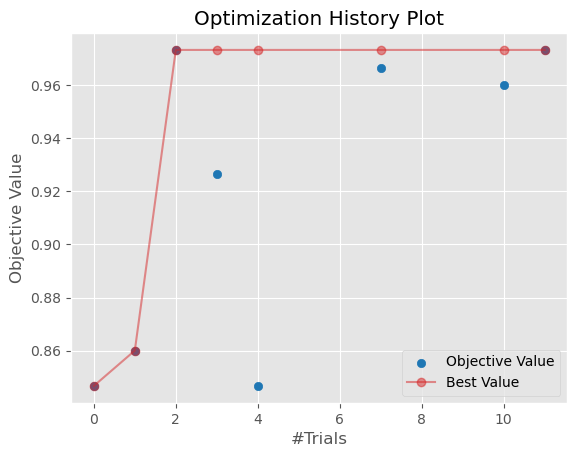tuner.plot_intermediate_scores()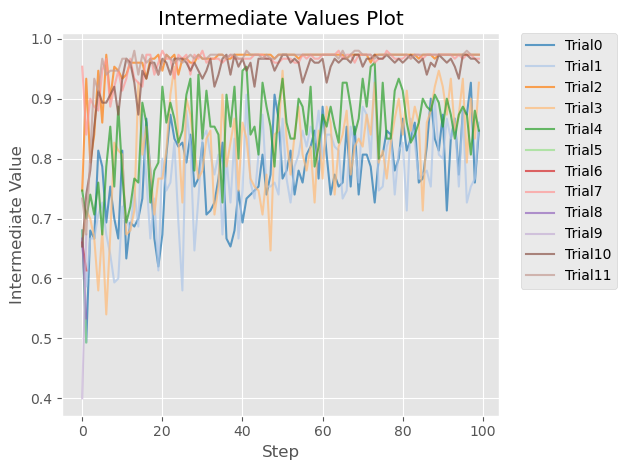tuner.plot_contour_scores(params=['penalty', 'alpha'])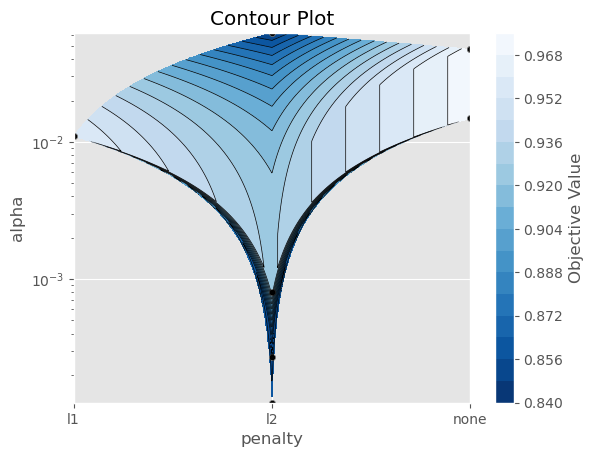tuner.plot_parallel_coordinate_scores(params=['penalty', 'alpha'])tuner.plot_edf_scores()tuner.plot_param_importance()

Waiting for more trials before evaluating the param importance.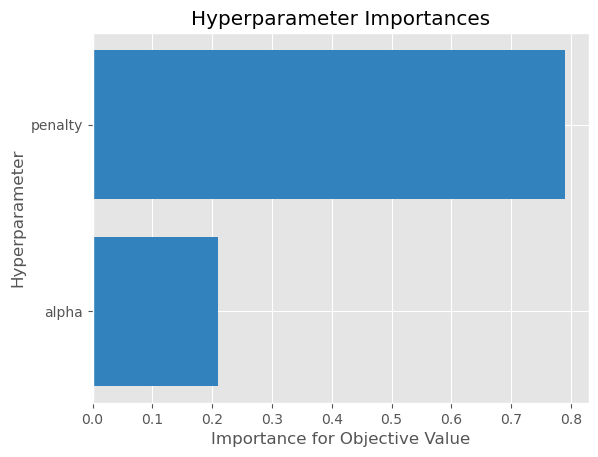Defining a Custom Search Space and Score

Instead of using a perfunctory or detailed strategy, define a custom search space strategy.

The next cell, creates a LogisticRegression() model instance then defines a custom search space strategy for the three LogisticRegression() hyperparameters, C, solver, and max_iter parameters.

You can define a custom scoring parameter, see Optimizing a scikit-learn Pipeline() though this example uses the standard weighted average $$F_1$$, f1_score.

tuner = ADSTuner(LogisticRegression(),
strategy = {'C': LogUniformDistribution(low=1e-05, high=1),
'solver': CategoricalDistribution(['saga']),
'max_iter': IntUniformDistribution(500, 2000, 50)},
scoring=make_scorer(f1_score, average='weighted'),
cv=3)
tuner.tune(X, y, exit_criterion=[NTrials(5)], synchronous=True, loglevel=logging.WARNING)


Changing the Search Space Strategy

You can change the search space in the following three ways:

• Remove existing hyperparameters

• Modify the range of existing non-categorical hyperparameters

Note: You can’t change the distribution of an existing hyperparameter or make any changes to a hyperparameter that is based on a categorical distribution. You need to initiate a new ADSTuner object for those cases. For more detailed information, review the adstuner_search_space_update.ipynb notebook.

The next cell switches to a detailed strategy. All previous values set for C, solver, and max_iter are kept, and ADSTuner infers distributions for the remaining hyperparameters. You can force an overwrite by setting overwrite=True.

tuner.search_space(strategy='detailed')

{'C': LogUniformDistribution(low=1e-05, high=10),
'solver': CategoricalDistribution(choices=['saga']),
'max_iter': IntUniformDistribution(low=500, high=2000, step=50),
'dual': CategoricalDistribution(choices=[False]),
'penalty': CategoricalDistribution(choices=['elasticnet']),
'l1_ratio': UniformDistribution(low=0, high=1)}


Alternatively, you can edit a subset of the search space by changing the range.

tuner.search_space(strategy={'C': LogUniformDistribution(low=1e-05, high=1)})

{'C': LogUniformDistribution(low=1e-05, high=1),
'solver': CategoricalDistribution(choices=['saga']),
'max_iter': IntUniformDistribution(low=500, high=2000, step=50),
'dual': CategoricalDistribution(choices=[False]),
'penalty': CategoricalDistribution(choices=['elasticnet']),
'l1_ratio': UniformDistribution(low=0, high=1)}


Here’s an example of using overwrite=True to reset to the default values for detailed:

tuner.search_space(strategy='detailed', overwrite=True)

{'C': LogUniformDistribution(low=1e-05, high=10),
'dual': CategoricalDistribution(choices=[False]),
'penalty': CategoricalDistribution(choices=['elasticnet']),
'solver': CategoricalDistribution(choices=['saga']),
'l1_ratio': UniformDistribution(low=0, high=1)}

tuner.tune(X, y, exit_criterion=[NTrials(5)], synchronous=True, loglevel=logging.WARNING)


Optimizing a scikit-learn Pipeline

The following example demonstrates how the ADSTuner hyperparameter optimization engine can optimize the sklearn Pipeline() objects.

You create a scikit-learn Pipeline() model object and use ADSTuner to optimize its performance on the iris dataset from sklearn.

The dataset is then split into X and y, which refers to the training features and the target feature respectively. Again, applying a train_test_split() call splits the data into training and validation datasets.

X, y = load_iris(return_X_y=True)
X = pd.DataFrame(data=X, columns=["sepal_length", "sepal_width", "petal_length", "petal_width"])
y = pd.DataFrame(data=y)

numeric_features = X.select_dtypes(include=['int64', 'float64', 'int32', 'float32']).columns
categorical_features = y.select_dtypes(include=['object', 'category', 'bool']).columns

y = preprocessing.LabelEncoder().fit_transform(y)

num_features = len(numeric_features) + len(categorical_features)

numeric_transformer = Pipeline(steps=[
('num_imputer', SimpleImputer(strategy='median')),
('num_scaler', StandardScaler())
])

categorical_transformer = Pipeline(steps=[
('cat_imputer', SimpleImputer(strategy='constant', fill_value='missing')),
('cat_encoder', ce.woe.WOEEncoder())
])

preprocessor = ColumnTransformer(
transformers=[
('num', numeric_transformer, numeric_features),
('cat', categorical_transformer, categorical_features)
]
)

pipe = Pipeline(
steps=[
('preprocessor', preprocessor),
('feature_selection', SelectKBest(f_classif, k=int(0.9 * num_features))),
('classifier', LogisticRegression())
]
)


You can define a custom score function. In this example, it is directly measuring how close the predicted y-values are to the true y-values by taking the weighted average of the number of direct matches between the y-values.

def custom_score(y_true, y_pred, sample_weight=None):
score = (y_true == y_pred)
return np.average(score, weights=sample_weight)

score = make_scorer(custom_score)


Again, you instantiate the ADSTuner() object and use it to tune the iris dataset:

ads_search = ADSTuner(
pipe,
scoring=score,
strategy='detailed',
cv=2,
random_state=42)



The ads_search tuner can provide useful information about the tuning process, like the best parameter that was optimized, the best score achieved, the number of trials, and so on.

ads_search.sklearn_steps

{'classifier__C': 9.47220908749299,
'classifier__dual': False,
'classifier__l1_ratio': 0.9967712201895031,
'classifier__penalty': 'elasticnet',
'classifier__solver': 'saga'}

ads_search.best_params

{'C': 9.47220908749299,
'dual': False,
'l1_ratio': 0.9967712201895031,
'penalty': 'elasticnet',
'solver': 'saga'}

ads_search.best_score

0.9733333333333334

ads_search.best_index

12

ads_search.trials.head()

number value datetime_start datetime_complete duration params_classifier__C params_classifier__dual params_classifier__l1_ratio params_classifier__penalty params_classifier__solver user_attrs_mean_fit_time user_attrs_mean_score_time user_attrs_mean_test_score user_attrs_metric user_attrs_split0_test_score user_attrs_split1_test_score user_attrs_std_fit_time user_attrs_std_score_time user_attrs_std_test_score state
0 0 0.333333 2021-04-21 20:04:24.353482 2021-04-21 20:04:24.484466 0 days 00:00:00.130984 0.001479 False 0.651235 elasticnet saga 0.011303 0.002970 0.333333 custom_score 0.333333 0.333333 0.003998 0.000048 0.000000 COMPLETE
1 1 0.953333 2021-04-21 20:04:24.494040 2021-04-21 20:04:24.580134 0 days 00:00:00.086094 0.282544 False 0.498126 elasticnet saga 0.008456 0.003231 0.953333 custom_score 0.946667 0.960000 0.000199 0.000045 0.006667 COMPLETE
2 2 0.333333 2021-04-21 20:04:24.587609 2021-04-21 20:04:24.669303 0 days 00:00:00.081694 0.003594 False 0.408387 elasticnet saga 0.007790 0.002724 0.333333 custom_score 0.333333 0.333333 0.000228 0.000074 0.000000 COMPLETE
3 3 0.333333 2021-04-21 20:04:24.677784 2021-04-21 20:04:24.760785 0 days 00:00:00.083001 0.003539 False 0.579841 elasticnet saga 0.007870 0.002774 0.333333 custom_score 0.333333 0.333333 0.000768 0.000146 0.000000 COMPLETE
4 4 0.333333 2021-04-21 20:04:24.768813 2021-04-21 20:04:24.852988 0 days 00:00:00.084175 0.000033 False 0.443814 elasticnet saga 0.008013 0.003109 0.333333 custom_score 0.333333 0.333333 0.000185 0.000486 0.000000 COMPLETE
ads_search.n_trials

20
`

References